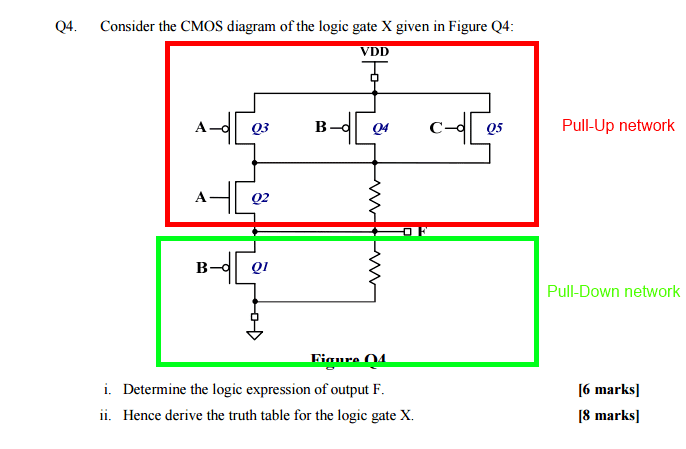# Pull Up/Pull Down Circuit

• Engineering

## Homework Statement

Based on a circuit diagram below

None really

## The Attempt at a Solution

So I was going through some past exams papers and encountered this question.
However at the top it says it's a Pull-down circuit.

Now I know that pull-down circuits utilize nmos transistors and pull-up circuits utilize pmos transistors, so the diagram coincides with that convention.
However the circuit is established between Vdd and the output...so it's setup as a pull-up circuit(atleast to me). So if it's a pull-up circuit then the soln would be basically to convert that diagram to Boolean form.

But if it is in fact pull-down circuit then the soln would be the dual of the above.

Which should I take it as in order to work out the soln.

#### Attachments

•pulluppulldown.png
48.2 KB · Views: 575

CWatters
Homework Helper
Gold Member
Tricky. I'd do the other questions in the exam first and come back to this one if I had time!

I think you have to point out that the circuit appears inconsistent or at least unconventional. If it's a pull-down then Vdd must be the lower voltage supply rail (0V) where as normally Vdd is the higher supply voltage (typically 5V). In addition circuits are conventionally drawn with the higher voltage rails at the top of the page and lower voltages at the bottom, so if this is a pull-down then it's been draw "upside down". On the other hand the FETs are shown as N-Type which would be consistent with a pull down.

I would hope that if you state your assumptions and solve it either way you should get the marks.

Thanks man
I guess I would work with it assuming it's a pull down circuit.

This is a pretty stupid question to bring on a final exam btw :|

CWatters
Homework Helper
Gold Member
It can be done if the threshold voltage is small. If VB is constrained to be ≥ 0V then the lowest VF will go is 0V + VTH. If VB can go below 0V then VF can approach 0V.

PS: NMOS logic came before CMOS. NMOS used N-Type FETs as pull-up "resistors".

Oh so the pull down cct in the second qn would just consist of the pmos transistor B?

But then that works out be not B and its not the dual of the pull up above...or am I approaching it wrong?

CWatters
Homework Helper
Gold Member
Oh so the pull down cct in the second qn would just consist of the pmos transistor B?

I assume you mean Q1 rather than "transistor B".

There is also a pull down resistor shown in parallel with Q1.

Yes Q1 sorry lol

So because of the two pull down resistors i'm assuming that the entire circuit is made up of two separate pull down ccts.
Going with this assumption... my soln is

(~Q3+~Q4+~Q5)*(Q1Q2)

CWatters
Homework Helper
Gold Member
Two pull down resistors?Last edited:
CWatters
Homework Helper
Gold Member
Going with this assumption... my soln is
(~Q3+~Q4+~Q5)*(Q1Q2)

I don't follow that. Instead of writing the logic expression in terms of Q1 to Q5 you should be using the inputs A,B and C.

For the pull down networks...normally the soln is the NOT of the expression of the pull down network so going with the pic you sent...the circuit's final expression would be ~~B = B?

But then that wouldn't be the Dual of the pull up circuit...this is where i'm confused :(

CWatters
Homework Helper
Gold Member
This is how I approached it...

I started by looking to see what had to happen to make F true (logic 1).

Looking at the pull down circuit it's clear that F can only be true if Q1 is off so B must be true. So the equation for F is going to start something like...

F = B AND (something else)

To make F true something must also pull it up (otherwise there is a resistor to pull it down/false). So I then looked at the pull up circuit to see what had to happen to pull F up. That gives you the "something else" expression. Have a think about that and the effect of the resistor in the pull up circuit. For example what happens if Q2 is off and Q5 is on? Is that sufficient to make F a logic 1?

CWatters